# 堆排序（大堆）

其实堆的空间结构实际上就是一棵完全二叉树，不过堆有它自己的限定条件。大堆就是树中每个父亲节点都要比孩子节点大，并且两个兄弟之间是没有大小区分的。小堆与之相反，就是每个父亲节点都要比孩子节点小。

堆的空间结构虽然是个完全二叉树，但是这样的结构也是我们为了好理解才想出来的，实际上堆是通过数组的下标进行相应的排序，因为完全二叉树中的结点的编号可以算出来，这样就能与数组进行对应。

1)初始化堆：将R[1..n]构造为堆；也就是构造成一棵完全二叉树

2)将当前无序区的堆顶元素R同该区间的最后一个记录交换，然后将新的无序区调整为新的堆。

因此对于堆排序，最重要的两个操作就是构造初始堆和调整堆，其实构造初始堆事实上也是调整堆的过程，只不过构造初始堆是对所有的非叶节点都进行调整。

1.堆  堆实际上是一棵完全二叉树，其任何一非叶节点满足性质：  Key[i]<=key[2i+1]&&Key[i]<=key[2i+2]或者Key[i]>=Key[2i+1]&&key>=key[2i+2]  即任何一非叶节点的关键字不大于或者不小于其左右孩子节点的关键字。  堆分为大顶堆和小顶堆，满足Key[i]>=Key[2i+1]&&key>=key[2i+2]称为大顶堆，满足 Key[i]<=key[2i+1]&&Key[i]<=key[2i+2]称为小顶堆。由上述性质可知大顶堆的堆顶的关键字肯定是所有关键字中最大的，小顶堆的堆顶的关键字是所有关键字中最小的。

下面举例说明：

给定一个整形数组a[]={16,7,3,20,17,8}，对其进行堆排序。

首先根据该数组元素构建一个完全二叉树，得到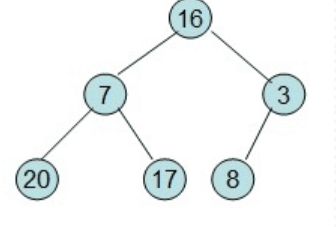3)由于交换后新的堆顶R可能违反堆的性质，因此需要对当前无序区(R1,R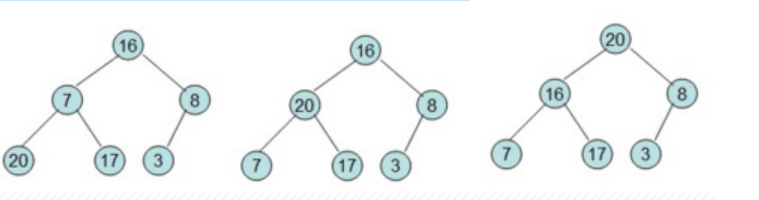20和16交换后导致16不满足堆的性质，因此需重新调整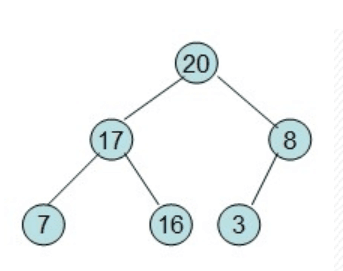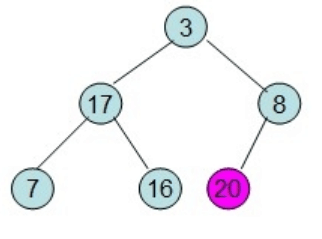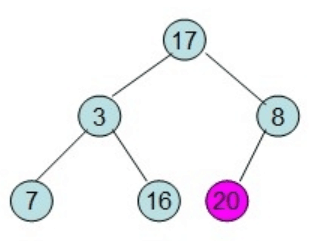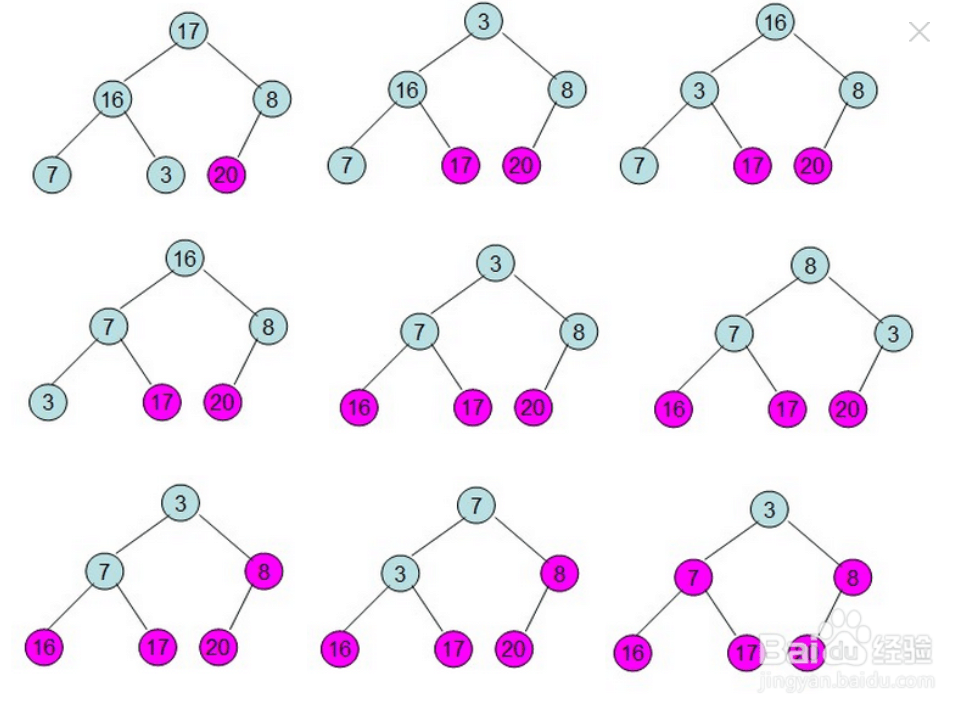#include<stdio.h>

#define LEFT nRootID*2+1
#define RIGHT nRootID*2+2

void Adjust(int arr[],int nLength,int nRootID)
{
while(1)
{
//两个孩子
if(RIGHT < nLength)
{
//比较两个孩子大小
if(arr[LEFT] > arr[RIGHT])
{
//大的和父亲比较
if(arr[LEFT] > arr[nRootID])
{
arr[LEFT] = arr[LEFT]^arr[nRootID];
arr[nRootID] = arr[LEFT]^arr[nRootID];
arr[LEFT] = arr[LEFT]^arr[nRootID];

//被交换位置成为下次调整位置
nRootID = LEFT;
continue;
}
break;
}
else
{
if(arr[RIGHT] > arr[nRootID])
{
arr[RIGHT] = arr[nRootID]^arr[RIGHT];
arr[nRootID] = arr[nRootID]^arr[RIGHT];
arr[RIGHT] = arr[nRootID]^arr[RIGHT];

nRootID = RIGHT;
continue;
}
break;
}
}
else if(LEFT < nLength)
{
if(arr[LEFT] > arr[nRootID])
{
arr[LEFT] = arr[LEFT]^arr[nRootID];
arr[nRootID] = arr[LEFT]^arr[nRootID];
arr[LEFT] = arr[LEFT]^arr[nRootID];

//被交换位置成为下次调整位置
nRootID = LEFT;
continue;
}
break;
}
else
{
break;
}
}
}

void Adjust2(int arr[],int nLength,int nRootID)
{
int MAX;

for(MAX = LEFT;MAX < nLength; MAX = LEFT)
{
//两个孩子
if(RIGHT < nLength)
{
if(arr[MAX] < arr[RIGHT])
{
MAX = RIGHT;
}
}

if(arr[MAX] > arr[nRootID])
{
arr[MAX] = arr[MAX] ^ arr[nRootID];
arr[nRootID] = arr[MAX] ^ arr[nRootID];
arr[MAX] = arr[MAX] ^ arr[nRootID];

nRootID = MAX;
continue;
}
else
{
break;
}
}
}
void HeapSort(int arr[],int nLength)
{
if(arr == NULL || nLength <= 0)return;

int i;
//建初始堆
//从最后一个父亲节点开始调整
for(i = nLength/2-1;i>=0;i--)
{
Adjust2(arr,nLength,i);
}

//排序
for(i = nLength-1;i>0;i--)
{
//交换
arr = arr ^ arr[i];
arr[i] = arr ^ arr[i];
arr = arr ^ arr[i];

//调整跟节点
Adjust2(arr,i,0);
}
}

int main()
{
int arr[] = {10,2,45,3,8,108,5,7};
HeapSort(arr,sizeof(arr)/sizeof(arr));

int i;
for(i = 0;i<sizeof(arr)/sizeof(arr);i++)
{
printf("%d ",arr[i]);
}
printf("\n");
return 0;
}